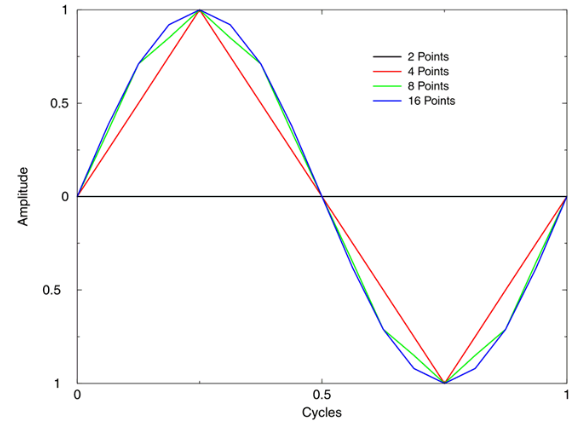# How meshing relates to wavelength in OnScale

Meshing in OnScale is not automatic, unlike most finite element analysis (FEA) programs. OnScale gives users full control over the mesh, thus a mesh must be created before allocating materials. Generally this is very straightforward as most geometries in acoustic applications can be very well represented by a fine structured mesh.

The  element size, which we typically refer to as box, is one of the key components to obtaining accurate results.

In order to determine the size of box, the smallest wavelength must be calculated by dividing the lowest velocity in the model by the highest frequency of interest (λ = v / f). This is then used with the number of elements per wavelength to determine the element size.

In some cases, the lowest material velocity doesn't have to be used if you are not concerned with resolving particular wave modes in specific materials.

The following is a code snippet of how a mesh would be defined in OnScales Analyst mode:

```c frequency and mesh
symb freqint = 1e6                   /* frequency of interest
symb freqdamp = \$freqint             /* set damping frequency to frequency of operation
symb vel = 1500                      /* minimum material velocity in model
symb wavelength = \$vel / \$freqint    /* calculate smallest wavelength to discretised
symb nepw = 15                       /* number of elements per wavelength
symb box = \$wavelength / \$nepw       /* calculate element size for meshing
```

With the smallest wavelength calculated, more elements per wavelength (nepw) can be used to increase accuracy, however, this MUST be traded off against additional computational times. For example, in a 3D model, doubling the amount of elements per wavelength will multiply the model size by a factor of 8 as there are 3 axes (XYZ) to consider.Accuracy is subjective and is up to the user to determine what is required to obtain the best solution. At a minimum, we recommend using 15 elements per wavelength as a starting point but all users should be performing mesh convergence studies to understand how meshing is affecting their results.